Select Page

# Maths 12 Science CBSE Probability Answers for MCQ in Hindi

Maths 12 Science CBSE Probability Answers for MCQ in Hindi to enable students to get Answers in a narrative video format for the specific question.

Expert Teacher provides Maths 12 Science CBSE Probability Answers for MCQ through Video Answers in Hindi language. This video solution will be useful for students to understand how to write an answer in exam in order to score more marks. This teacher uses a narrative style for a question from Probability not only to explain the proper method of answering question, but deriving right answer too.

Please find the question below and view the Answer in a narrative video format.

Question:

## Similar Questions from CBSE, 12th Science, Maths, Probability

Question 1 : If E and F are two events such that,, Find P(not E and not F). (View Answer Video)

Question 2 : If P(A)=0.8, P(B)=0.5 and=0.4, find. (View Answer Video)

Question 3 : If P(A)=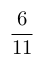, P(B)=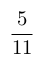and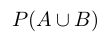=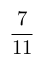, find. (View Answer Video)

Question 4 : Compute P(A/B) if P(B) =0.5 and P(A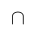B)=0.32. (View Answer Video)

### Application of Derivatives

Question 1 : What is the maximum value of the function sin x+ cos x? (View Answer Video)

Question 2 : Find the approximate change in volume V of a cube of side x meters caused by increasing the side by 1%. (View Answer Video)

Question 3 : Equation of normal to the curve x+y=x^y where it cuts x-axis; is (View Answer Video)

Question 4 : If,then the approximate value of f(3.02) is _________. (View Answer Video)

Question 5 : Find the maximum profit that a company can make if the profit function is.(View Answer Video)

### Differential Equations

Question 1 : Find the solution of the differential equation(View Answer Video)

Question 2 : Solve the differential equation(View Answer Video)

Question 3 : Write the degree of the differential equation(View Answer Video)

Question 4 : Write the degree of the differential equation :(View Answer Video)

Question 5 : Write the differential equation representing the family of curves y = mx, where m is an arbitrary constant. (View Answer Video)

### Matrices

Question 1 : Find the transpose of the matrix:. (View Answer Video)

Question 2 : LetFind A + B. (View Answer Video)

Question 3 : Find the value of Y, ifand. (View Answer Video)

Question 4 : Find the value of x, if. (View Answer Video)

Question 5 : LetFind A - B. (View Answer Video)